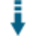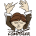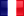# PHP Random.Org API: Generate random values using random.orgDownload .zip
 InfoView files (12)Download .zip Reputation Support forum Blog Links
 Last Updated Ratings Unique User Downloads Download Rankings 2022-10-21 (11 months ago)Not enough user ratings Total: 138 All time: 9,193 This week: 204Version License PHP version Categories randomorg 1.0.1 GNU General Publi... 5.5 PHP 5, Text processing, Web services
Description

Author

This class can generate random values using random.org.

It can send requests to the random.org Web site server to retrieve random values of different types. Currently it can retrieve:

- Random string of text and binary data
- Float and integer numbers
- Sequence of integer numbersPerformance Level
Name: Classes: Christian Vigh `` 32 packages by Christian VighFrance 57 138 10 in France81 in FranceInnovation awardNominee: 20x

Winner: 3x

Details

# INTRODUCTION

This site allows you to retrieve true random numbers, which are different from the ones returned by pseudo-random generators such as the builtin rand() and mt_rand() PHP functions.

Pseudo-random generators are usually implemented by a distribution function with a formula that uses prime numbers. To sum up a little bit, if you call a pseudo-number generator function 100 times to return you a number between 1 and 100, then you will obtain numbers between 1 and 100, in an order that appears to be random.

The seed (the initial value used for the computation of the first pseudo-random number) has a direct influence on the order of the results.

Of course, as you might guess, you cannot obtain true random numbers from deterministic methods. I'm not aware of any pseudo-random number generator that could return you the same consecutive value more than once. Of course, these are handy methods that can help you to test your code with some "random" data, but they are subject to caution when talking about cryptography aspects or even password generators, because they are deterministic.

This is where the random.org* website (and theRandomOrg PHP class) enter into action. The consecutive values that you will be able to retrieve from the site through this class have clearly no mathematical relashionship between them. They are simply random.

## HOW DOES IT WORKS ?

The random.org site offers an API to freely retrieve random values, with quota restrictions. Data can be fetched by using one of the following methods :

• You are not registered. In this case, you can issue simple HTTP requests to retrieve data. This is described as the old API on the random.org site. Your current IP address is used by the site to enforce the quota restrictions.
• You have registered yourself ; in this case, you can use the JSON/RPC API (also called the new API), and your credentials will be used by the site to enforce the quota restrictions.

The current version of the RandomOrg class uses the old API and does not require you to register on the site.

## RESTRICTIONS

The services offered by random.org are mostly free ; however, you must obey to a certain number of usage rules that are described here : https://www.random.org/clients/.

To sum up :

• During your very first connection to the site (which is "authenticated" through your ip address), you are attributed a quota of 1 000 000 bits. Retrieve a byte value, your current quota will be subtracted by 8 bits ; 32 bits for an integer value, 64 bits for floating-point values, and 8 bits per character when you retrieve random strings.
• Every 24 hours, you are credited with 200 000 additional bits, but your quota can never exceed 1 000 000 bits.
• Don't use multi-threaded simultaneous requests
• If you need many numbers, issue ONE single request to get them all, instead of issuing multiple requests for retrieving only one number at a time (remember that there is an HTTP request to process each time)
• Use a long timeout between two requests, or risk your requests to be discarded
• Regularly check your quota (such a request does not consume any bits of it) to determine whether it did not reach a negative number (or take the risk of being temporarily banned from the site).
• Use your email address as the user agent string for HTTP requests ; this will allow the site to drop you a mail in case of problems

Such restrictions may seem drastic, but remember it's a free service...

## WHAT IS THE ROLE OF THE RandomOrg CLASS ?

In such a context, the RandomOrg class helps you to :

• Retrieve random byte, integer, floating-point or string values
• Check for you the current status of your quota and throw an exception if you fall below it

For the latter case, the RandomOrg class distinguishes between two types of requests :

• Large requests : they are aimed at retrieving a large number of random values. The class will check your current quota and will throw an exception if there is a risk that it falls below the value of the RandomOrg::QUOTA\_REJECT\_LARGE\_REQUESTS constant (which is expressed in bits).
• Small requests : Even if you reached your quota for large requests, you can still issue small requests as long as they are not greater than RandomOrg::SMALL\_REQUEST\_SIZE bits and that your current quota does not fall below RandomOrg::QUOTA\_REJECT\_SMALL\_REQUESTS bits.

Note that you cannot retrieve more than RandomOrg::MAX\_VALUES (10000) at once.

# REFERENCE

## Quota usage

All the methods retrieving random values affect your current quota ; the rules are the following :

• Your current quota will be subtracted by the amount of bits you requested (each method description gives you a method for calculating the size of your request)
• If your request size exceeds RandomOrg::SMALL\_REQUEST\_SIZE bits, then the function will check that your current quota remains above RandomOrg::QUOTA\_REJECT\_LARGE\_REQUESTS bits after executing the request. If your request size is such that it would make you fall behind this value, or if your quota is already less than this value, then every data-retrieval method will return false instead of an array of values.
• The same rules applies for requests whose size is less than or equal to RandomOrg::SMALL\_REQUEST\_SIZE bits. In this case, the lower limit of your quota is RandomOrg::QUOTA\_REJECT\_SMALL\_REQUESTS bits.
• You can use the GetQuota() method to retrieve your current quota whenever you want. Calling this method does not affect your current quota.

## METHODS

### CONSTRUCTOR

``````\$random = new RandomOrg ( \$user_agent = null ) ;
``````

Instantiates an object for accessing the random.org site, using the specified user agent string for http accesses.

If not specified, the value used for the user agent string will be that of the static variable RandomOrg::\$GlobalUserAgent. It is highly recommended that you put your email address here, so that the random.org site can contact you in case of problems.

Instantiating a RandomOrg object automatically retrieves your current quota from the site, that you can later get using the GetQuota() method.

### \$array = \$random -> GetBytes ( \$count )

Retrieve a sequence of \$count random bytes as an array.

The \$count parameter must be between 1 and RandomOrg::MAX_BYTE_VALUES.

The total size of the request which will be deduced from your current quota is : \$count 8 bits.

### \$float = \$random -> GetFloat ( \$decimal\_places = RandomOrg::MAX\_DECIMAL\_PLACES ) ;

Retrieves one single floating-point value. You can specify the number of decimal places you want, up to RandomOrg::MAX\_DECIMAL\_PLACES (20).

The returned value will be in the range 0..1, using the number of decimal places you specified.

The total size of the request which will be deduced from your current quota is : 64 bits.

IMPORTANT NOTE : This method is provided here only for convenience reasons, when all you need is one floating-point value. You mustn't use this method in a loop to retrieve values one by one. Should this be the case, use the GetFloats() method instead, specifying the number of values you really want to retrieve.

### \$array = \$random -> GetFloats ( \$count, \$decimal_places = RandomOrg::MAX_DECIMAL_PLACES ) ;

Retrieves \$count floating-point values. You can specify the number of decimal places you want, up to RandomOrg::MAX\_DECIMAL\_PLACES (20).

The method returns an array of floating-point values, in the range 0..1, using the number of decimal places you specified..

The total size of the request which will be deduced from your current quota is : count \* 64 bits.

### \$integer = \$random -> GetInteger ( \$min = RandomOrg::MIN\_INTEGER\_VALUE, \$max = RandomOrg::MAX\_INTEGER\_VALUE ) ;

Retrieves one single integer value. You can specify a range restriction for the \$min and max parameters.

By default, the returned value will be in the range RandomOrg::MIN\_INTEGER\_VALUE (-1,000,000,000) and RandomOrg::MAX\_INTEGER\_VALUE (+1,000,000,000)

The returned value will be in the range \$min..\$max.

The total size of the request which will be deduced from your current quota is : 32 bits.

IMPORTANT NOTE : This method is provided here only for convenience reasons, when all you need is one integer value. You mustn't use this method in a loop to retrieve values one by one. Should this be the case, use the GetIntegers() method instead, specifying the number of values you really want to retrieve.

### \$array = \$random -> GetIntegers ( \$count, \$min = RandomOrg::MIN\_INTEGER\_VALUE, \$max = RandomOrg::MAX\_INTEGER\_VALUE ) ;

Retrieves \$count integer values. You can specify a range restriction for the \$min and max parameters.

By default, the returned value will be in the range RandomOrg::MIN\_INTEGER\_VALUE (-1,000,000,000) and RandomOrg::MAX\_INTEGER\_VALUE (+1,000,000,000).

The method returns an array of integer values, in the range \$min..\$max.

The total size of the request which will be deduced from your current quota is : \$count \* 32 bits.

Retrieves one single random password of the specified length.

This method is an alias for :

``````\$password 	=  \$random -> GetString ( \$length, RandomOrg::STRING_ALPHA ) ;
``````

The total size of the request which will be deduced from your current quota is : \$length \* 8 bits.

IMPORTANT NOTE : This method is provided here only for convenience reasons, when all you need is one random password string value. You mustn't use this method in a loop to retrieve values one by one. Should this be the case, use the GetPasswords() method instead, specifying the number of values you really want to retrieve.

### \$array = \$random -> GetPasswords ( \$count, \$length ) ;

Retrieves \$count random passwords of the specified length.

This method is an alias for :

``````\$password_array	=  \$random -> GetStrings ( \$count, \$length, RandomOrg::STRING_ALPHA ) ;
``````

The total size of the request which will be deduced from your current quota is : \$count \\$length\ 8 bits.

### \$quota = \$random -> GetQuota ( \$current = true, \$ip = null ) ;

Retrieves your current quota, in bits.

When the \$current parameter is true, the method uses the last quota that the RandomOrg class has computed for you. When false, it will issue an http request to the random.org site to retrieve the actual quota.

Note that issuing an http request for retrieving your quota does not affect your quota...

### \$array = \$random -> GetSequence ( \$min, \$max ) ;

Returns an array of integer values in the range \$min..\$max, arranged in a truly random order.

The values \$min and \$max must be in the range RandomOrg::MIN\_INTEGER\_VALUE (-1,000,000,000) and RandomOrg::MAX\_INTEGER\_VALUE (+1,000,000,000).

The value \$max - \$min + 1 must not exceed RandomOrg::MAX\_SEQUENCE\_RANGE (10000).

The total size of the request which will be deduced from your current quota is : ( \$max - \$min + 1 ) \* 32 bits.

### \$string = \$random -> GetString ( \$length, \$options = RandomOrg::STRING\_ALPHA ) ;

Retrieves a random string of length \$length, using the generation options specified by the \$options parameter (see the Constants section for a list of available options).

The \$length parameter must not exceed RandomOrg::MAX\_SRING\_LENGTH (20).

The total size of the request which will be deduced from your current quota is : \$length \* 8 bits.

### \$array = \$random -> GetStrings ( \$count, \$length, \$options = self::STRING\_ALPHA ) ;

Retrieves an array of count random strings of length \$length, using the generation options specified by the \$options parameter (see the Constants section for a list of available options).

The \$length parameter must not exceed RandomOrg::MAX\_SRING\_LENGTH (20).

The total size of the request which will be deduced from your current quota is : \$count \\$length\ 8 bits.

### Informational functions

The set of methods described below provides you with information related to the last executed query. They all have a \$global parameter which determines the level of information you want to retrieve :

• When false (the default), the methods return information about the last query executed by the instance of the RandomOrg class you are using
• When true, they return information about the last executed query. This can be useful only if you are managing multiple instances of the RandomOrg class, and are only interested in the most recent results.

#### \$query = \$random -> GetQuery ( \$global = false ) ;

Returns the HTTP query that was built for the latest request that has been issued.

#### \$time = \$random -> GetQueryTime ( \$global = false ) ;

Returns the time where the last HTTP query was executed, as a Unix timestamp.

#### \$text = \$random -> GetResult ( \$global = false ) ;

Returns the raw text results obtained through the last HTTP request.

#### \$elapsed = \$random -> GetElapsed ( \$global = false ) ;

Returns the elapsed time, in milliseconds, of the last executed HTTP request.

#### \$time = \$random -> GetResultTime ( \$global = false ) ;

Returns the time where the results of the last HTTP request were received, as a Unix timestamp.

#### \$type = \$random -> GetType ( \$global = false ) ;

Returns the type of the last executed query ; it can be one of the following values :

• 'byte' : the last executed query was performed by the GetBytes() method
• 'integer' : GetInteger() or GetIntegers()
• 'float' : GetFloat() or GetFloats()
• 'sequence' : GetSequence()

#### \$array = \$random -> GetQueryInfo ( \$global = false ) ;

This method is a combination of all of the above ; it returns an associative array with the following entries :

• type
• query
• query-time
• result
• result-time
• elapsed
• quota

## PROPERTIES

This class does not contain any public properties.

## CONSTANTS

The following constants are defined for enforcing the management of your daily quota :

• QUOTA\_PER\_DAY : Max quota, in bits, authorized by the random.org site (1,000,000)
• QUOTA\_REJECT\_LARGE\_REQUESTS : When this quota has been reached (50000), large requests, ie requests greater than QUOTA\_SMALL\_REQUEST\_SIZE bits (1024) will be rejected and the corresponding Getxxx() method will return false. Note however that small requests will still continue to be served, until the QUOTA\_REJECT\_SMALL\_REQUESTS* value will be reached.
• QUOTA\_REJECT\_SMALL\_REQUESTS : Small requests (less than QUOTA\_SMALL\_REQUEST\_SIZE bits) will continue to be served until this quota limit (5000) has been reached.
• QUOTA\_SMALL\_REQUEST\_SIZE : The size in bits (1024) which determines whether the QUOTA\_SMALL\_REQUEST\_SIZE or QUOTA\_LARGE\_REQUEST\_SIZE limit should be used to enforce quota checking.

The following constants specifies the limits for some HTTP requests :

• MIN\_INTEGER\_VALUE (-1000000), MAX\_INTEGER\_VALUE (+1000000) : Min and max values for retrieving integers using the GetInteger() and GetIntegers() methods.
• MAX\_STRING\_LENGTH (20) : Maximum string length that can be retrieved for random passwords and strings
• MAX\_SEQUENCE\_RANGE (10000) : Maximum range of values that can be processed by the GetSequence() method
• MAX\_DECIMAL\_PLACES (20) : Maximum number of decimal places in the floating-point values returned by the GetFloat() and GetFloats() methods

The following constants give the limits on the number of values that can be retrieved in one shot :

• MAX\_PASSWORD\_VALUES (100) : Maximum number of random strings that can be retrieved at once by the GetPasswords() and *GetStrings() methods.
• MAX\_BYTE\_VALUES (16384): Maximum number of random bytes that can be retrieved at once by theGetBytes()* method.
• MAX\_VALUES (10000) : Maximum number of random values that can be retrieved by all the other methods (GetIntegers(), GetFloats() and GetSequence())

The following set of bits can be specified for the \$options parameter of the GetString() and *GetStrings() methods :

• STRING_DIGITS : The generated random string(s) will contain only digits.
• STRING_UPPER : The generated random string(s) will contain only uppercase letters.
• STRING_LOWER : The generated random string(s) will contain only lowercase letters.
• STRING_ALPHA : The generated random string(s) will contain letters (uppercase and lowercase) and digits.Files
File Role Descriptionexamples (8 files)LICENSE Lic. License textNOTICE Data Auxiliary dataRandomOrg.phpclass Class Class sourceREADME.md Doc. DocumentationFiles / examples
File Role Descriptionexamples.inc.php Aux. Auxiliary scriptgetbytes.php Example Example scriptgetfloats.php Example Example scriptgetintegers.php Example Example scriptgetpasswords.php Example Example scriptgetquota.php Example Example scriptgetsequence.php Example Example scriptgetstrings.php Example Example script

 All time: 9,193 This week: 204About usAdvertise on this siteSite mapNewsletterStatisticsSite tipsPrivacy policyContact
For more information send a message to `info at phpclasses dot org`.